# How to Generate Random Positive and Negative Numbers in Excel?

Random data is a type of data generated randomly. In this article, the users will learn how to generate random positive and negative numbers by using the predefined available Excel methods, and kutool. Random data can be used for any purpose such as creating a sample data set or evaluating results based on the dataset. This article contains four examples in which the first two examples will allow the user to generate random positive and negative numbers by using available formula methods, while the remaining two examples will allow the user to generate positive and negative numbers by using the kutools.

## Example 1: To generate a random positive number in Excel by using the predefined formula:

### Step 1:

To understand the process of generating the random positive number. Let’s consider an empty Excel sheet, with an active B2 cell.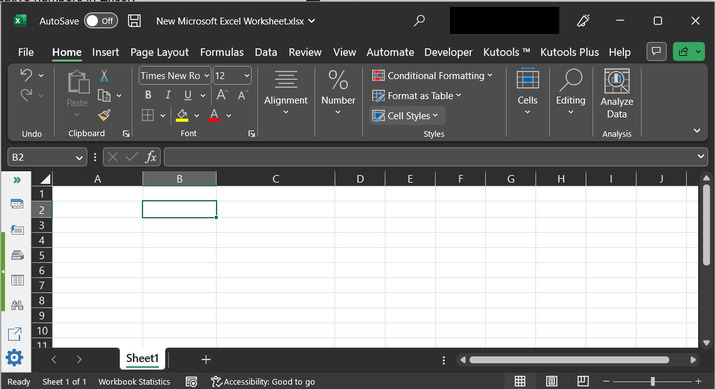### Step 2:

For this example, the user needs to use the “=RANDBETWEEN(X, Y)” method. For this method, the values of both the X and Y variables should be positive. Then only the generated number is guaranteed to be a positive random number.

For this example, let’s assume that the user wants to generate a positive random number between the range 1 and 100. To do so simply type the formula “=RANDBETWEEN(1,100)”.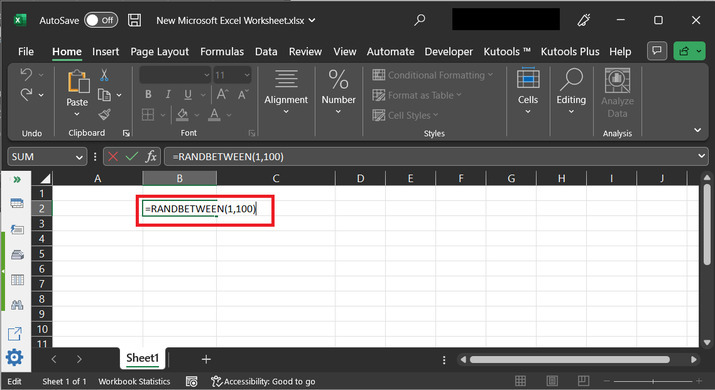### Step 3:

After typing the above-provided formula, Press the “Enter” key. This will display a positive random number on the active cell. Consider the below-depicted image, to understand the output properly.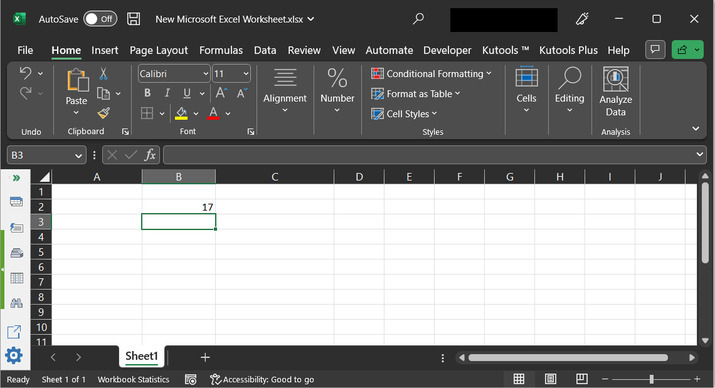## Example 2: To generate a random negative number in Excel by using the predefined formula:

### Step 1:

To understand the process of generating the random negative number. It is better to consider an empty Excel sheet, with an active B2 cell.### Step 2:

It is clear if the user wants to generate a negative random number then the provided range must be negative. For this case, let’s assume that user type “=RANDBETWEEN(-100,-1)”.

Here, the provided range is from -100 to -1.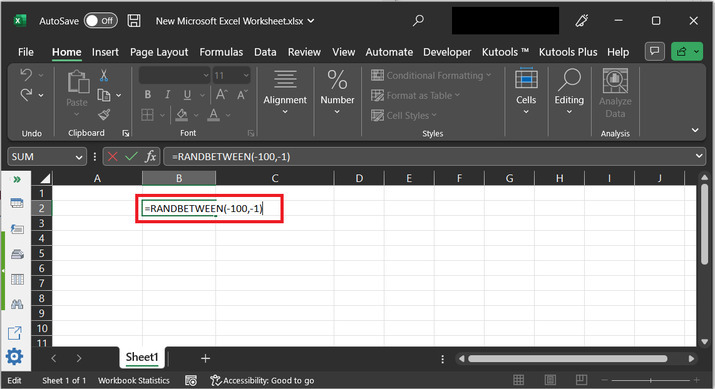### Step 3:

Press the “Enter” key, and a negative random value will appear on the console as shown below: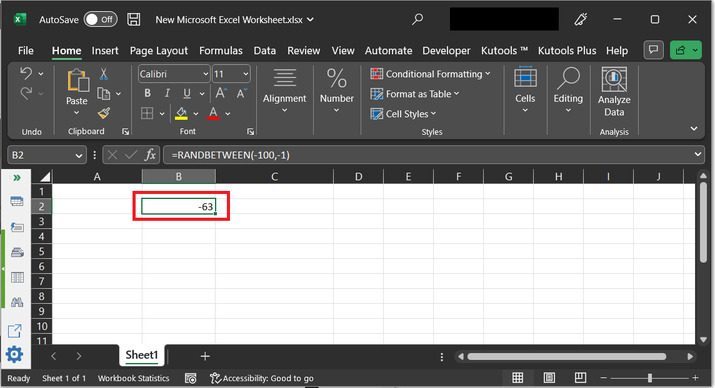## Example 3: To generate a random positive number in Excel by using kutools:

### Step 1:

To understand the process of generating a random number. Let’s consider an empty Excel sheet, with B2 active cell.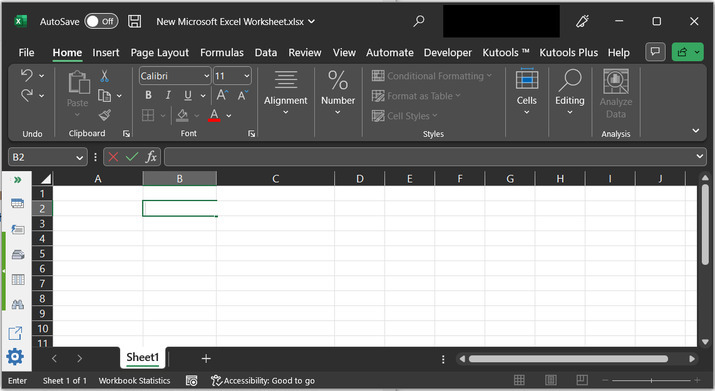### Step 2:

First of all, go to the “Kutools” tab and then choose the “Editing” option. After that select the “Insert” option, and choose the “Insert Random Data” option. Consider below shown highlighted factors to understand all the required chosen operations carefully.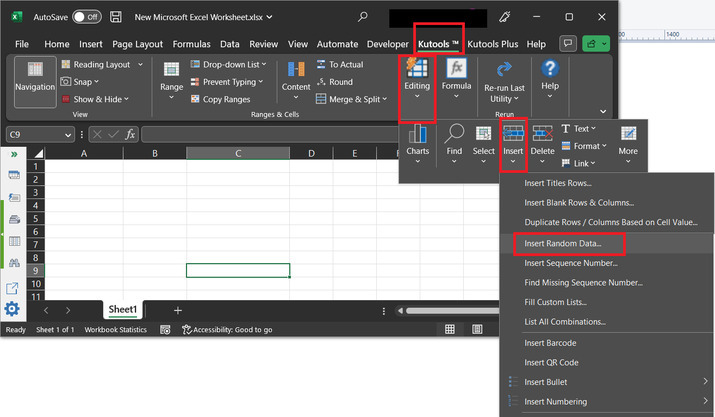### Step 3:

This will open the “Insert Random Data” dialog box, as provided below. In this dialog box, choose the “Integer” tab as highlighted in the below image.

Note − Integer type values are required for this task.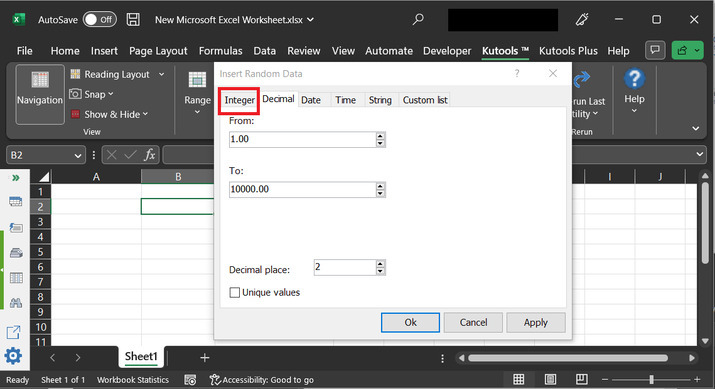### Step 4:

Go to the “From” and “To” label to choose the range. In the opened dialog box, type “1” in the From label, and “100” to the “To ” label, and finally click on “Ok” button.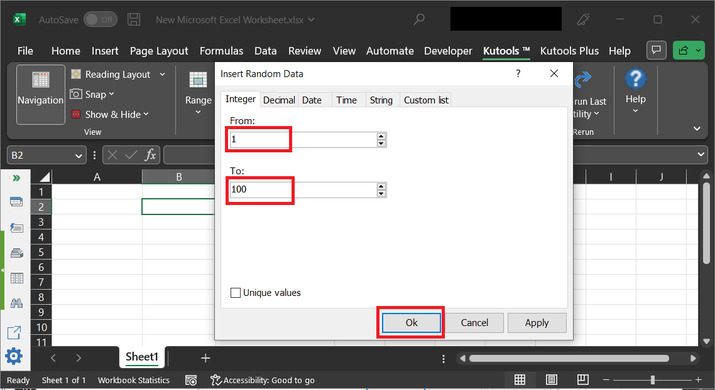### Step 5:

After the selecting of the “OK” button. The required positive random number will be printed on the active cell within the Excel sheet. Consider the below-depicted image for output: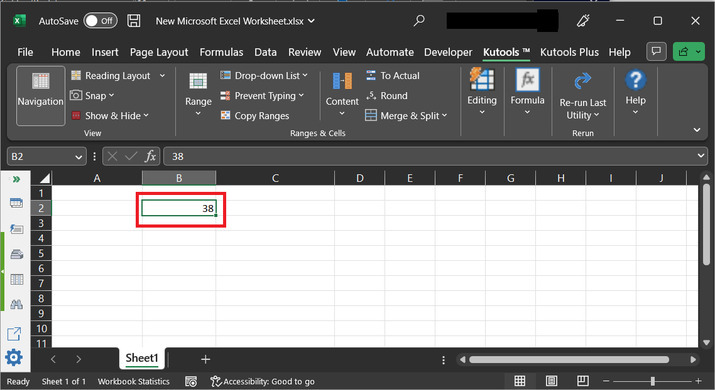## Example 4: To generate a random negative number in Excel by using kutool:

### Step 1:

To understand the process of generating a random number. Let’s consider an empty Excel sheet, with an active B2 cell, and Go to the kutool tab and select the “Insert Random Data” option.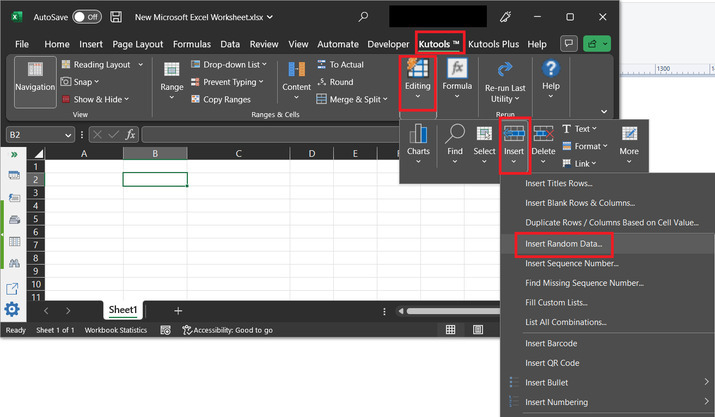### Step 2:

In the From and To labels enter the negative range values. For this example, let’s enter -100 to the “From” label, and -20 to the “To” label. Finally, click on the “Ok” button.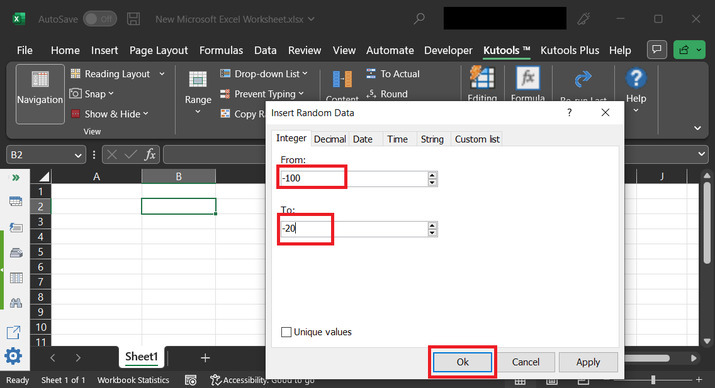### Step 3:

This will display the below generated numbers on console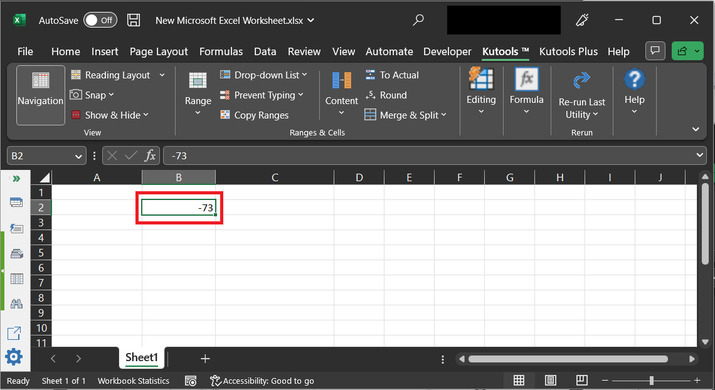## Conclusion:

This article allows users to understand the process to generate positive and negative random numbers. The first two examples involve the usage of the RANDOM() method to create random positive and negative numbers, while the third and fourth examples use the “Kutool” to generate the same.

Updated on: 17-Apr-2023

376 Views